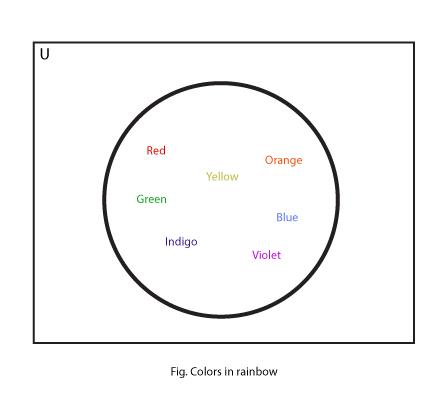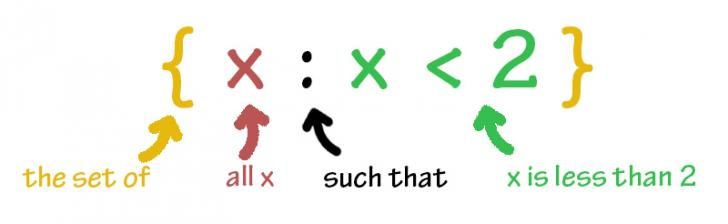# Set Notation

A set is a collection of distinct objects(elements) which have common property. For example, red, blue, and green are colors. When the elements are considered collectively, set is formed.

The elements in a set can be represented in a number of ways, some of which are more useful for mathematical treatment and others for general understanding. These different methods of describing a set are called set notations.

## Set Notations

Sets are among the most useful ways of visualizing relationships between different classes of data. Concept of sets helps us to deal with several relationships in functions, Differential Geometry and many other fields.

To perform these functions, sets need certain notation for uniformity in representation. If we get familiar to them, it will be easier for us to understand sets and their relationships. Such notations help us to study set relations in a uniform and universal way and makes the representations easier.

Sets can be defined in three ways:

### 1. Statement Method

In this method, a set is denoted by a brief sentence describing the common property (well defining the set) of the elements. The sentence is then enclosed with a pair of curly brackets ‘{}’ Eg:

• The set of planets in our Solar system is denoted as:
`{ Planets in our Solar System }`
• The set of BRICS nations is denoted as:
`{ BRICS nations }`
• The set of colors of rainbow is denoted as:
`{ Colors of Rainbow }`

### 2. Roster Form or Tabular Form

In this method, the elements are listed and enclosed with the curly brackets. Eg:

• The set of planets in our Solar system is denoted as:
`{ Mercury, Venus, Earth, Mars, Jupiter, Saturn, Uranus, Neptune }`
• The set of SAARC nations is denoted as:
`{ Nepal, India, Pakistan, Afghanistan, Bhutan, Bangladesh, Maldives, Sri Lanka }`
• The set of colors of rainbow is denoted as:
`{ Red, Orange, Yellow, Green, Blue, Indigo, Violet }`

REMEMBER: ORDER OF LIST IS NOT IMPORTANT. BUT, WE MUST AVOID REPETITIONS AND OMISSIONS.### 3. Set Builder Notation

Set-builder notation is a mathematical shorthand where we state all the properties that an element of the set should possess.

e.g. if we want to describe a set of numbers from 1 to 7, we know that each number in the set should have the following properties

1. It should be a natural number
2. Its value should be greater than 0 and less than 8

These two properties are sufficient to describe each element of the set. The mathematical formula or notation so formed that completely describes the set is also termed as set abstraction, set comprehension or the definition of the set’s intention.

This system is used by denoting the elements by ‘x’ or any other variables. Then, the variable is defined by the rule we created earlier

`eg  A = {x: x is a planet in the Solar System}`

Let us consider a set C comprising up of the colors in the rainbow. The notation by way of Set Builder Notation System is:

```C = {x|x is a color in the rainbow.} or,
C = {x:x is a color in the rainbow.}```